EE(Exploitation & Exploration) 问题在计算广告/推荐系统中非常常见，甚至在更广义的范围上，任意决策问题都会牵涉到 EE 问题。简单来说，这个问题就是要解决的是在决策时到底是根据已有经验选择最优的策略(Exploitation)，还是去探索一些新的策略来提升未来的收益(Exploration)。本文主要介绍解决这个问题的三种比较常见的方法：随机方法，UCB 方法，Thompson sampling 方法，侧重于方法的具体流程和基本思想。

## MAB 建模

EE 问题一般会通过 MAB(Multi-Armed Bandit) 进行建模, 如下所示，所有 arm 就是每次决策中可作出的选择，拉下某个 arm 表示作出了相应的选择。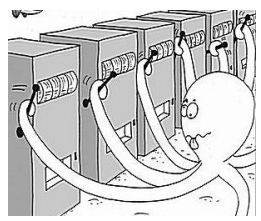MAB 符号化表述如下

1. MAB 可表示为一个二元组 <$A, R$>
2. $A$ 表示为一系列可能的动作, $R(r|a)$ 则表示给定动作下的奖赏的分布，
3. 每一时刻根据给定策略从 $A$ 选择动作 $a_t$, 同时环境根据分布 $R(r|a)$ 生成奖赏 $r_t$
4. 目标是最大化奖赏之和 $\sum_{t=1}^T r_t$

UCB 方法和 Thompson sampling 方法均可解决这两类问题，UCB 解决 Bernoulli Bandit 的方法有 UCB1，UCB2 等，解决 Contextual Bandit 的方法有 LinUCB 等；而 Thomson Sampling 解决 Bernoulli Bandit 时采用了 Bernoulli 分布和 Beta 分布，解决 Contextual Bandit 时采用了两个正态分布。后面会详细介绍这些方法。

## 随机方法

### $\epsilon$-greedy

$\epsilon$-greedy 是一种最简单的随机方法，原理很简单：每次决策时，以 1 - $\epsilon$ 的概率选择最优的策略，以 $\epsilon$ 的概率随机选择任意一个策略; 并且在每次做出决策获取到真实的 reward 后更新每个决策的收益情况（用于选择最优策略）。伪代码实现可参考 Multi-Armed Bandit: epsilon-greedy

$\epsilon$-greedy 存在着以下几个比较显著的问题

(1) $\epsilon$ 是个超参数，设置过大会导致决策随机性过大，设置过小则会导致探索性不足
(2) $\epsilon$-greedy 策略运行一段时间后，对各个 arm 的收益情况有所了解，但没有利用这些信息，仍然不做任何区分地随机 exploration（会选择到明显较差的item）
(3) $\epsilon$-greedy 策略运行一段时间后，但仍然花费固定的精力去 exploration，浪费了本应该更多进行 exploitation 机会

$$\epsilon = \frac{1}{1 + \log(m+1)}$$

### Softmax

$$p_i = \frac{e^{\mu_i/T}}{\sum_{j=0}^{n} e^{\mu_j/T}}(i=0, 1,…., n)$$

## UCB 方法

### UCB1

UCB1 方法的理论基础是 Hoeffding’s inequality，该不等式的定义如下

$$p(|E[X] - \overline{X}| \le \delta) \ge 1 - 2e^{-2n\delta^2}$$

UCB1 方法中将 $\delta$ 设为如下公式，公式中的 $N$ 表示目前所有 arm 试验的总次数，$n$ 表示某个 arm 的实验次数

$$\delta = \sqrt{\frac{2\ln{N}}{n}}$$

UCB1 方法的流程如下，该图摘自 Optimism in the Face of Uncertainty: the UCB1 Algorithm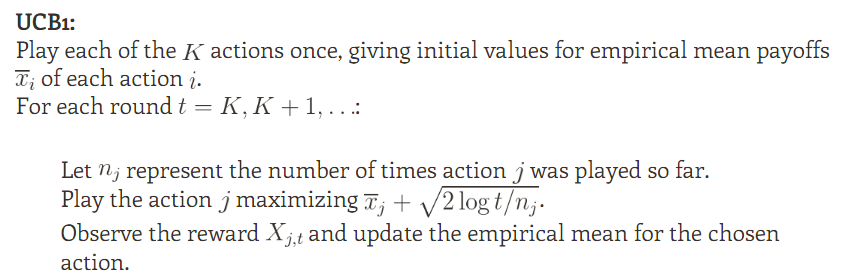• Bernstein’s inequality
• Empirical Bernstein’s inequality
• Chernoff inequality
• Azuma’s inequality
• …….

### UCB2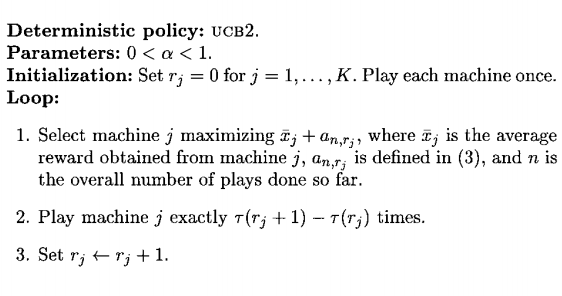$$a_{n,r} = \sqrt{\frac{(1+\alpha)\ln(ne/\tau(r))}{2\tau(r)}}$$

$$\tau(r) = \lceil (1+\alpha)^r\rceil$$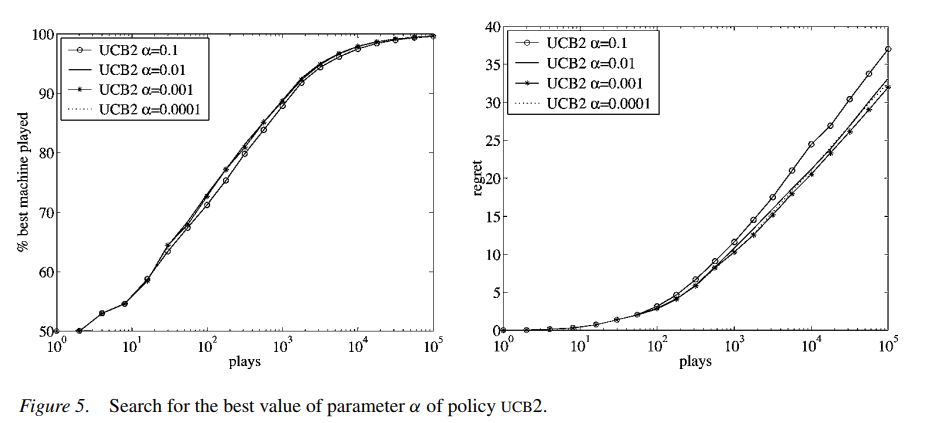### LinUCB

UCB1 中推到出 bound 的 Hoeffding’s inequality 不能直接应用到 LinUCB 中，而关于 linear regression 的 bound 最早是在这篇论文 Exploring compact reinforcement-learning representations with linear regression 中提出的，这里不详细展开具体的证明过程了。提出 LinUCB 的论文 A Contextual-Bandit Approach to Personalized News Article Recommendation 直接采用了这一结论，其表达形式，如下所示

$$p(|x_j^T\theta_j - E(r|x_j)| \le \alpha \sqrt{x_j^T(D_j^TD_j+I)^{-1}x_j}) \ge 1- \delta$$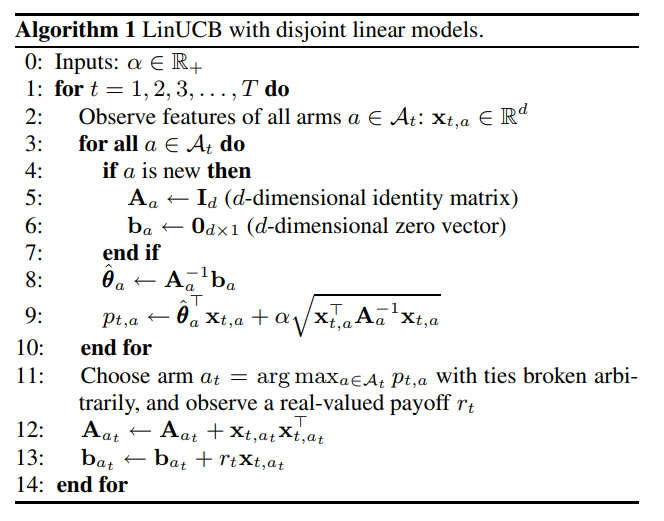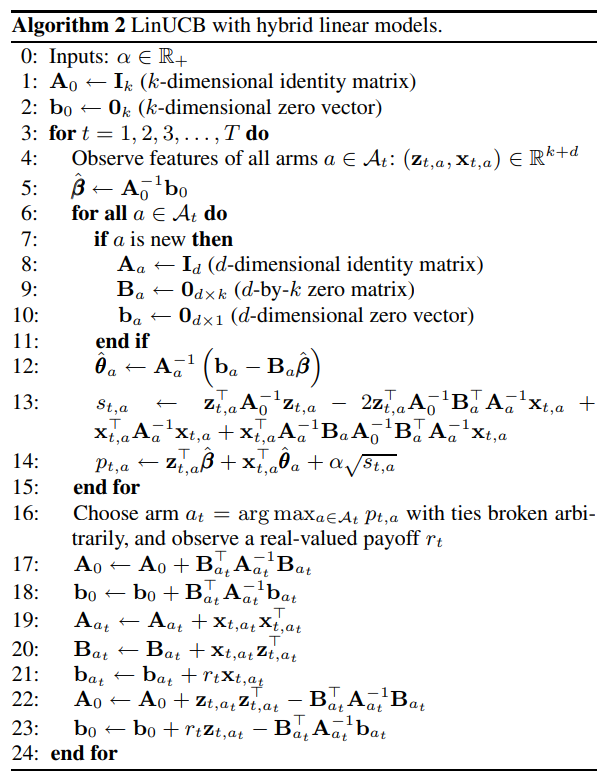## Thompson sampling 方法

### Bernoulli Bandit

$p(reward | \theta) \sim Bernoulli(\theta)$

UCB 方法中的 UCB1 和 UCB2 都是通过简单的历史统计得到 $\overline {x}_j$ 来表示 $\theta$ 的，但是贝叶斯学派则认为 $\theta$ 服从着一个特定的分布，根据贝叶斯公式有

$$p(\theta|reward) = \frac{p(reward|\theta) p{(\theta)}}{p(reward)} \propto p(reward|\theta) p{(\theta)} ＝Bernoulli(\theta) p(\theta)$$

$p(\theta|reward)$ 表示根据观察到的实验收益情况更新的后验概率，且由于似然 $p(reward|\theta)$ 为伯努利分布，为了保持共轭便于计算；先验分布 $p(\theta)$ 选择为了 Beta 分布，即 $Beta(\alpha, \beta)$，而两个分布相乘 $Bernoulli(\theta)*Beta(\alpha, \beta)$ 会得到一个新的 Beta 分布, 简单来说，就是

• 当 $Bernoulli(\theta)$ 的结果为1，则会得到 $Beta(\alpha + 1, \beta)$
• 当 $Bernoulli(\theta)$ 的结果为0，则会得到 $Beta(\alpha, \beta + 1)$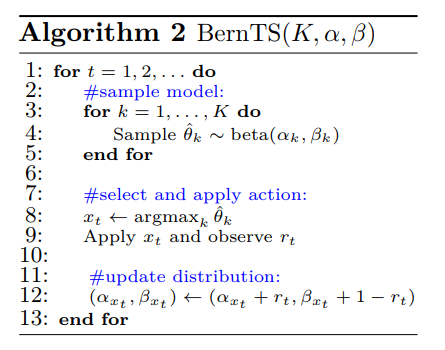### Contextual Bandit

$$p(\theta|reward) \propto p(reward|\theta) p{(\theta)}$$

$$B(t) = I_d + X^TX$$

$$\mu(t) = B(t)^{-1}(\sum_{\tau = 1}^{t-1} x_{\tau}^Tr_{\tau})$$

## 小结

UCB 采用的是频率学派的思想，计算的收益值是历史收益加上一个 bound，可以认为历史收益是 Exploitation，而 bound 则是 Exploration；而 Thompson Sampling 方法中每个 arm 的收益值会从一个分布中产生，没有明显的 Exploitation 和 Exploration，但也可以认为从分布中较大概率产生的值是 Exploitation ，而较小概率产生的值是 Exploration。另外，文中重点是介绍这些方法的具体做法，更多关于这些方法的理论基础和数学推导可参考下面这些文献。